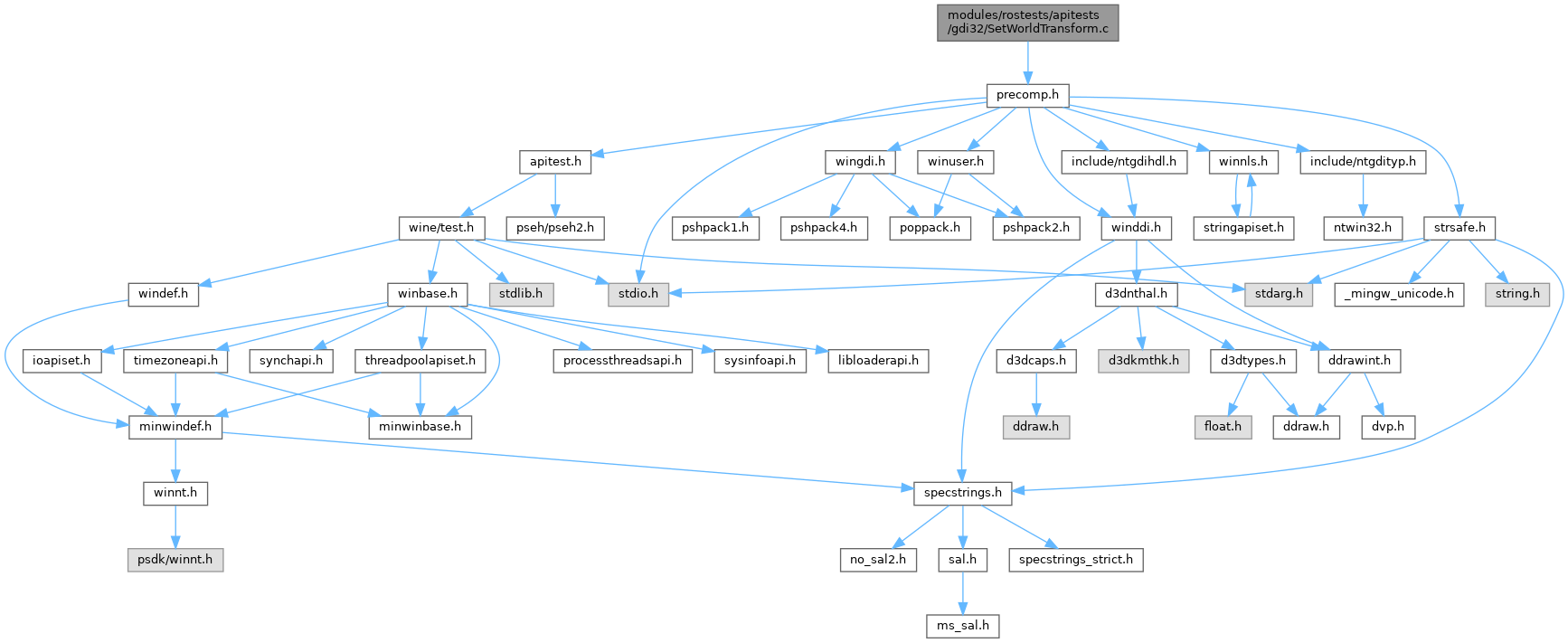ReactOS  0.4.15-dev-1152-g6c94e4f
SetWorldTransform.c File Reference
`#include "precomp.h"`
Include dependency graph for SetWorldTransform.c:Go to the source code of this file.

## Functions

void Test_SetWorldTransform ()

START_TEST (SetWorldTransform)

## ◆ START_TEST()

 START_TEST ( SetWorldTransform )

Definition at line 121 of file SetWorldTransform.c.

122 {
124 }
void Test_SetWorldTransform()

## ◆ Test_SetWorldTransform()

 void Test_SetWorldTransform ( )

Definition at line 11 of file SetWorldTransform.c.

12 {
13  HDC hdcScreen, hdc;
14  XFORM xform;
15  BOOL result;
16  //PGDI_TABLE_ENTRY pEntry;
17  //DC_ATTR* pdcattr;
18
19  /* Create a DC */
20  hdcScreen = GetDC(NULL);
21  hdc = CreateCompatibleDC(hdcScreen);
22  ReleaseDC(NULL, hdcScreen);
24
25  /* Set identity transform */
26  xform.eM11 = 1;
27  xform.eM12 = 0;
28  xform.eM21 = 0;
29  xform.eM22 = 1;
30  xform.eDx = 0;
31  xform.eDy = 0;
32  result = SetWorldTransform(hdc, &xform);
33  ok(result == 1, "SetWorldTransform should succeed\n");
34
35  /* Set eM11 to 0 */
36  xform.eM11 = 0;
37  result = SetWorldTransform(hdc, &xform);
38  ok(result == 0, "SetWorldTransform should fail\n");
39
40  /* Set eM22 to 0 */
41  xform.eM11 = 1;
42  xform.eM22 = 0;
43  result = SetWorldTransform(hdc, &xform);
44  ok(result == 0, "SetWorldTransform should fail\n");
45
46  /* Set values that result in the determinant being 0 */
47  xform.eM11 = 2;
48  xform.eM12 = 3;
49  xform.eM21 = 4;
50  xform.eM22 = 6;
51  result = SetWorldTransform(hdc, &xform);
52  ok(result == 0, "SetWorldTransform should fail\n");
53
54  /* Small modification to make the determinant != 0 */
55  xform.eM12 = (FLOAT)3.0001;
56  result = SetWorldTransform(hdc, &xform);
57  ok(result == 1, "SetWorldTransform should succeed\n");
58
59  /* Set values that result in the determinant being 0 due to rounding */
60  xform.eM11 = 1;
61  xform.eM12 = (FLOAT)0.9999999;
62  xform.eM21 = (FLOAT)1.0000001;
63  xform.eM22 = 1;
64  ok(xform.eM12 != (FLOAT)1.0, "xform.eM12 shouldn't be 1.0\n");
65  ok(xform.eM21 != (FLOAT)1.0, "xform.eM21 shouldn't be 1.0\n");
66  ok(xform.eM12 * xform.eM21 != (FLOAT)1.0, "xform.eM12 * xform.eM21 shouldn't be 1.0\n");
67  result = SetWorldTransform(hdc, &xform);
68  ok(result == 0, "SetWorldTransform should fail\n");
69
70  /* Test world transform (should be unchanged by previous failure) */
71  result = GetWorldTransform(hdc, &xform);
72  ok(result == 1, "GetWorldTransform should succeed\n");
73  ok(xform.eM11 == 2, "xform.eM11 should be 2\n");
74  ok(xform.eM12 == (FLOAT)3.0001, "xform.eM12 should be 3.0001\n");
75  ok(xform.eM21 == 4, "xform.eM21 should be 4\n");
76  ok(xform.eM22 == 6, "xform.eM22 should be 6\n");
77
78  /* Set smallest possible values */
79  xform.eM11 = 1.17549435e-38f;
80  xform.eM12 = 0;
81  xform.eM21 = 0;
82  xform.eM22 = 1.17549435e-38f;
83  ok(xform.eM11 != (FLOAT)0.0, "xform.eM11 shouldn't be 0.0\n");
84  ok(xform.eM22 != (FLOAT)0.0, "xform.eM22 shouldn't be 0.0\n");
85  ok(xform.eM11 * xform.eM22 != (FLOAT)0.0, "xform.eM12 * xform.eM21 shouldn't be 0.0\n");
86  result = SetWorldTransform(hdc, &xform);
87  ok(result == 1, "SetWorldTransform should succeed\n");
88
89  /* Test world transform */
90  result = GetWorldTransform(hdc, &xform);
91  ok(result == 1, "GetWorldTransform should succeed\n");
92  ok(xform.eM11 > 0, "xform.eM11 should not be 0\n");
93  ok(xform.eM12 == 0, "xform.eM12 should be 0\n");
94  ok(xform.eM21 == 0, "xform.eM21 should be 0\n");
95  ok(xform.eM22 > 0, "xform.eM22 should not be 0\n");
96
97  xform.eM11 = 0;
98  xform.eM12 = 1;
99  xform.eM21 = 1;
100  xform.eM22 = 0;
101  result = SetWorldTransform(hdc, &xform);
102  ok_int(result, 1);
103
104  xform.eM11 = 1;
105  xform.eM12 = 1;
106  xform.eM21 = 1;
107  xform.eM22 = 1;
108  result = SetWorldTransform(hdc, &xform);
109  ok_int(result, 0);
110
111  result = GetWorldTransform(hdc, &xform);
112  ok_int(result, 1);
113  ok(xform.eM11 == 0, "xform.eM11 should be 0\n");
114  ok(xform.eM12 == 1, "xform.eM12 should be 1\n");
115  ok(xform.eM21 == 1, "xform.eM21 should be 1\n");
116  ok(xform.eM22 == 0, "xform.eM22 should be 0\n");
117
118  DeleteDC(hdc);
119 }
#define FLOAT
Definition: i386-dis.c:516
HDC WINAPI GetDC(_In_opt_ HWND)
static HDC
Definition: imagelist.c:92
HDC WINAPI CreateCompatibleDC(_In_opt_ HDC hdc)
BOOL WINAPI SetWorldTransform(_In_ HDC, _In_ const XFORM *)
unsigned int BOOL
Definition: ntddk_ex.h:94
FLOAT eDx
Definition: wingdi.h:1724
int WINAPI ReleaseDC(_In_opt_ HWND, _In_ HDC)
FLOAT eM21
Definition: wingdi.h:1722
smooth NULL
Definition: ftsmooth.c:416
#define ok_int(expression, result)
Definition: atltest.h:134
FLOAT eM12
Definition: wingdi.h:1721
BOOL WINAPI GetWorldTransform(_In_ HDC, _Out_ LPXFORM)
Definition: coord.c:278
FLOAT eM11
Definition: wingdi.h:1720
HDC hdc
Definition: main.c:9
BOOL WINAPI DeleteDC(_In_ HDC)
#define f
Definition: ke_i.h:83
#define ok(value,...)
Definition: atltest.h:57
FLOAT eM22
Definition: wingdi.h:1723
FLOAT eDy
Definition: wingdi.h:1725
float FLOAT
Definition: typedefs.h:69
GLuint64EXT * result
Definition: glext.h:11304
int WINAPI SetGraphicsMode(_In_ HDC, _In_ int)
Definition: dc.c:1204